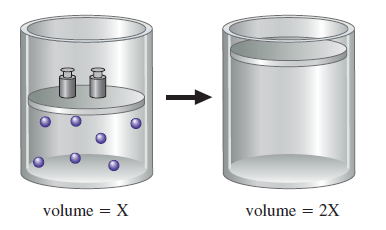# Problem: You have a gas in a container fitted with a piston, and you change one of the conditions of the gas such that a change takes place, as shown below:State three distinct changes you can make to accomplish this, and explain why each would work.

###### FREE Expert Solution

Recall that the ideal gas law is:

$\overline{){\mathbf{PV}}{\mathbf{=}}{\mathbf{nRT}}}$

The volume of a gas is related to the number of moles, pressure, gas constant, and temperatureThe value of R is constant

90% (468 ratings)###### Problem Details

You have a gas in a container fitted with a piston, and you change one of the conditions of the gas such that a change takes place, as shown below:State three distinct changes you can make to accomplish this, and explain why each would work.

Frequently Asked Questions

What scientific concept do you need to know in order to solve this problem?

Our tutors have indicated that to solve this problem you will need to apply the The Ideal Gas Law Applications concept. You can view video lessons to learn The Ideal Gas Law Applications. Or if you need more The Ideal Gas Law Applications practice, you can also practice The Ideal Gas Law Applications practice problems.

What professor is this problem relevant for?

Based on our data, we think this problem is relevant for Professor Leveritt's class at UIUC.

What textbook is this problem found in?

Our data indicates that this problem or a close variation was asked in Chemistry: An Atoms First Approach - Zumdahl Atoms 1st 2nd Edition. You can also practice Chemistry: An Atoms First Approach - Zumdahl Atoms 1st 2nd Edition practice problems.